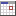# Simple interest calculator

This tool calculates how much interest will be charged on the principal only (initial amount that you invested) at certain interest rate for particular period of time. Simple interest calculations ignores the effects of compounding, so interest on interest is not included.

It can be calculated using the following equation:

FV = P + I
I = P * R * T

where:
FV = Future value
I = Interest amount
P = Principal amount
R = Interest rate (as a decimal, not in percentage)
T = Time period

Example 1:
The US federal government issued the following Treasury Notes (T-notes):
\$1000 T-note with a 3% annual rate, paid quartely, with a maturity in 5 years.

Question:
If you obtain a \$1000 T-note how much interest will you earn and what is your future value?
Interest amount I = 1000 * 0.03 * (4*5) = \$600
Future value FV = 1000 + 600 = \$1600

Example 2:
Jim borrowed \$2000 for 1.5 years at 0.1% per week simple interest.

Question:
How much interest is he charged and what is the total amount he has to pay back?
Interest amount I = 2000 * 0.001 * (1.5 * 52) = \$156
Total return amount FV = 2000 + 156 = \$2156

This simple interest calculator has many features:
• Calculate the future value (FV) and interest (I) using equation:

FV = P * (1 + R * T)     and     I = FV - P

• Calculate the principal (P) using equation:
 P = FV (1 + R * T)• Calculate the interest rate (R) using equation:
 R = FV - P P * T• Calculate the time period (T) using equation:
 T = FV - P P * R• Let you specify the interest rate for different time units:
Per day, week, two weeks, half month, month, two months, quarter, half year or year.

• Let you specify the time period in two ways:
• Method 1: Enter the time period by number of: days, weeks, bi-weeklies, semi-monthlies, months, bi-monthlies, quarters, semi-annuallies or years.
• Method 2: Enter a from date and to date. Both dates can also be included or excluded.

• The number of days in a year can be set to 360, 364, 365 or 366 days.

## Input simple interest calculator:

 Calculate:Future value (FV) and Interest (I)Principal (P)Interest rate (R)Time period (T) Future value (FV) or Interest (I)*:Future value (FV)Interest (I) Principal amount (P) *:Interest rate (R) *:%   per   dayweektwo weekshalf monthmonthtwo monthsquarterhalf yearyear Time period (T) *:daysweeksbi-weeklies (every two weeks)semi-monthlies (twice a month)monthsbi-monthlies (every two months)quarterssemi-annuallies (twice a year)years From / To dateFrom date:To date:Include From / To dateInclude from date: Include to date: Days in year:360364365366 * = required